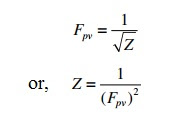Gas Compressibility Factor (Z):

• The compressibility factor, or gas deviation factor is a measure of how close a real gas is to an ideal gas.
• The compressibility factor is defined as the ratio of the gas volume at a given temperature and pressure to the volume the gas would occupy if it were an ideal gas at the same temperature and pressure.
• The compressibility factor is a dimensionless number which is close to 1.00.
• It is  a function of the gas gravity, gas temperature, gas pressure, and the critical properties of the gas.

## As an example, a particular natural gas mixture can have a compressibility factor equal to 0.87 at 1000 psia and 80°F.
## A Charts have been constructed that depict the variation of Z with the reduced temperature and reduced pressure.

Super-compressibility Factor (Fpv):

The super-compressibility factor (Fpv), which is related to the compressibility factor Zis defined as follows:There are several approaches to calculating the compressibility factor for a particular gas temperature T and pressure P.

Gas Compressibility Factor Formula:

The following methods are available to calculate the compressibility factor:

a) Standing-Katz method
b) Dranchuk, Purvis, and Robinson method
c) AGA method
d) CNGA method